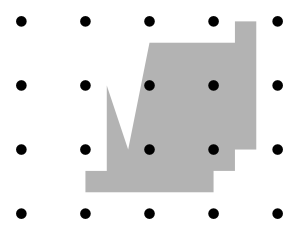### Problem Statement

Dissatisfied with the inconvenient spherical shape of the Earth, a group of map-makers have gone to Magrathea to obtain a custom-built planet. They decided to design their new planet to be flat and in the shape of a polygon. All coordinates will be in the Cartesian (x, y) plane.

In order to provide everyone on the planet with internet access, a wireless router will be placed at every lattice point that is inside the polygon (a lattice point is a point with integer coordinates). The map-makers decided to keep their job simple by choosing a polygon with no lattice points on its boundary.

Because the map-makers are highly rational people, they decided that the coordinates of all the vertices of the polygon would be fractions with a common denominator. The vertices of the polygon (in order), are given by the int[]s x and y, and by the common denominator denom. The ith vertex is (x[i]/denom, y[i]/denom).

Calculate and return the number of routers required.

### Definition

 Class: WifiPlanet Method: routersNeeded Parameters: int[], int[], int Returns: long Method signature: long routersNeeded(int[] x, int[] y, int denom) (be sure your method is public)

### Constraints

-x and y will contain the same number of elements.
-x and y will each contain between 3 and 50 elements, inclusive.
-Each element of x and y will be between 1 and 10^9, inclusive.
-No two vertices of the polygon will be the same.
-No two edges of the polygon (including their end-points) will intersect, with the exception that adjacent edges will intersect at their common end-point.
-No lattice point will lie on the boundary of the polygon.
-denom will be between 2 and 10^9, inclusive.

### Examples

0)

 `{1,7,3,3}` `{1,1,3,5}` `2`
`Returns: 2`
 This is illustrated in the image below:1)

 `{3,3,4,4,5,6,10,10,11,11,10,10,9,9}` `{1,2,2,6,3,8,8,9,9,3,3,2,2,1}` `3`
`Returns: 4`2)

 `{50,1000050,1000049}` `{2,1000002,1000003}` `100`
`Returns: 0`
3)

 `{32,32,64,8,15,1000,999}` `{1,10,10,48,48,47,1}` `16`
`Returns: 120`
4)

 `{1,1000000000,1000000000,1}` `{1,1,1000000000,1000000000}` `3`
`Returns: 111111110888888889`

#### Problem url:

http://www.topcoder.com/stat?c=problem_statement&pm=9733

#### Problem stats url:

http://www.topcoder.com/tc?module=ProblemDetail&rd=12182&pm=9733

bmerry

#### Testers:

PabloGilberto , legakis , Olexiy , ivan_metelsky

Geometry, Math